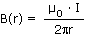# Force of Earth's magnetic field acting on a power line?

• ted182
In summary, a power line carrying 1400A through the Earth's magnetic field will experience a force of 5.6 N/m to the North, no force to the West, and a force of 2.8 N/m in a direction 30 degrees south of west. The force is calculated using the equation F = BILsin(theta) where theta is the angle between the current and the field. The Earth's magnetic field is assumed to be 4 x 10^-5 T and no other information is given, making it difficult to calculate the exact force without additional data.

## Homework Statement

Imagine a power line carrying 1400A through the Earth's magnetic field in each of the following directions. Per meter, what is the magnitude of the force acting on the line:
A. to the North?
B. to the West?
C. in a direction that points 30 degrees south of west?

## Homework Equationsand τ= B · I · L(1/2)W · sinθ (magnetic moment)

## The Attempt at a Solution

First off, I need to say that this is all the information supplied. Looking back in my lesson (Apex online learning AP Physics), I found one mention to the Earth's magnetic field as being 4· 10^-5 T, so I have to assume they want me to use this. No dimensions of the line are given, so my idea to find the magnetic moment sounds like it won't work. My other equation above is to calculate the field around the wire, but I would still need "r," the distance to the wire which is not specified. My only other though is that the force will be zero when the line is parallel to the Earth's magnetic field. I immediately assumed this would be when the line is running north, but now I am wondering if it could be west (which way does the Earth's field actually "run?"). So, I am stuck. The lack of useful equations and information I have been provided with makes it seem like the answer is perhaps easier to find than I believe, but I have no idea. Any thoughts?

Force on a current carrying wire is given by F = BILsin(theta) where theta is the angle between the current and the field.